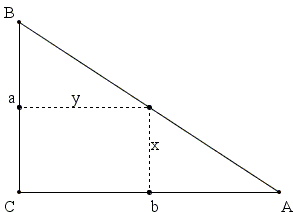[Fukagawa and Rothman, p. 117, problem 42] refer to Fujita Kagen's book Zoku Shinpeki Sanpo for the following problem

Take a point on the hypotenuse AB of the right triangle ABC. From that point draw perpendiculars to the legs of the triangles. The perpendiculars form a rectangle. Find the location of the point on the hypotenuse that maximizes the area of the rectangle.According to Fukagawa and Rothman, the problem was originally proposed in 1806 by Hotta Sensuke, a student of Fujita school, and written on a tablet hung in the Gikyosha shrine of Niikappugun, Hokkaido.

Solution

### References

1. H. Fukagawa, A. Rothman, Sacred Mathematics: Japanese Temple Geometry, Princeton University Press, 2008

## SangakuUsing the notations from the diagram, the task is to minimize the are S = xy.The configuration features several pairs of similar triangles that may be used to derive a relationship between x and y. In particular,

 yb
=
 (a - x)a

from which S = xy = (b/a)(ax - x²) and the problem reduces to finding maximum of the quadratic function f(x) = ax - x². As the leading coefficient of the function is -1, the graph of the function is a parabola with its "horns" extended downwards, thus insuring existence of a maximum.

I quote from [Fukagawa and Rothman, p. 139]:

Here is a traditional solution from the manuscript Solutions to Problems of Zoku Shinpeki Sanpo by Kitagawa Moko (1763-1833). In this case the traditional solution is pretty much what any calculus student would do (but see chapter 9.)

... Setting the derivative df/dx to 0 gives x = a/2 and, consequently, y = b/2.

As I am sure most of the calculus students will indeed find the maximum of function f(x) = ax - x² by equating the derivative to 0, I think it is worthwhile to quote from chapter 9 as mentioned by Fukagawa and Rothman above:

The question of differentiation in some sense is more difficult than that of integration because, although traditional Japanese mathematicians wrote volumes on integration techniques, they were virtually silent about how they took derivatives. One thing is certain: the Japanese did not have the concept of differentiation as we know it. In no traditional manuscript do we the fundamental formula for the derivative:

 limh → 0
 f(x + h) - f(x)h

Without this concept it is difficult or impossible to develop a formal theory of differentiation. Perhaps for this reason in the wasan differentiation was confined to finding the maximum and minimum of functions.

How did the traditional geometers do this? For quadratic equations, parabolas, one knows without calculus that the maximum or minimum is at the vertex of the parabola, and so for functions of the form y = ax² + bx + c, one can write down the answer xmin/max = -b/2a and ymin/max = y(xmin/max). By induction, one can do this for a few polynomials ...

My interpretation of the above is that, faced with the task of finding the maximum of the function obtained in the sangaku at hand, the traditional Japanese mathematicians would not, perhaps for the wrong reasons, calculate and subsequently equate to zero the derivative of quadratic functions. Unlike a majority of the present day calculus students, they would simply point out to a solution which is especially easy to surmise (and remember) for the function f(x) = ax - x² = x (a - x). Indeed, the parabola has symmetry around its axis which is known to pass through its vertex. The maximum (minimum) is, therefore, midway between the roots of the parabola which, in the case of the quadratic function f(x) = x (a - x), are obviously x = 0 and x = a, giving the maximum at x = (0 + a)/2 = a/2. Almost as easily one can obtain the same result by completing the square: ax - x² = -(x - a/2)² + a²/4.### A Sample of Optimization Problems II

• Mathematicians Like to Optimize
• Building a Bridge
• Building Bridges
• Optimization Problem in Acute Angle
• Geometric Optimization from the Asian Pacific Mathematical Olympiad
• Cassini's Ovals and Geometric Optimization
• Heron's Problem
• Optimization in Parallelepiped
• Matrices and Determinants as Optimization Tools: an Example
• An Inequality between AM, QM and GM
•